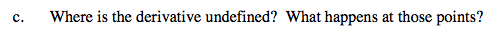### Home > CALC > Chapter 6 > Lesson 6.2.2 > Problem6-72

6-72.
1. ASTROID

2. The graph of x2/3 + y2/3 = 4 is called an "astroid." Homework Help ✎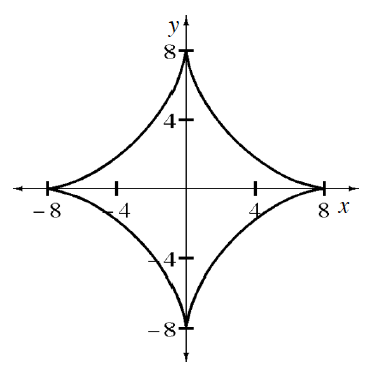1. Use implicit differentiation to find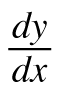.

2. Where does the astroid have a horizontal tangent? Explain.

3. Where is the derivative undefined? What happens at those points?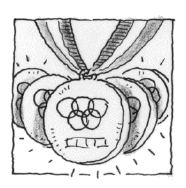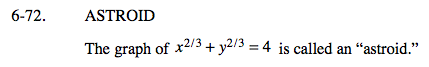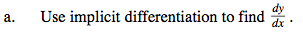$\frac{2}{3}x^{-1/3}+\frac{2}{3}y^{-1/3}y^\prime=0$

$\frac{2}{3}x^{-1/3}=-\frac{2}{3}y^{-1/3}y^\prime$

$y^\prime=-\frac{y^{1/3}}{x^{1/3}}$

$\frac{dy}{dx}=-\sqrt{\frac{y}{x}}$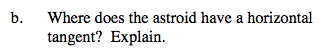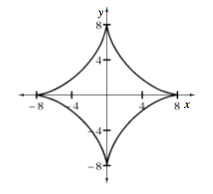Look at the derivative equation.

Now look at the graph. There are two coordinate points in which y = 0. Does the derivative exist at those points?